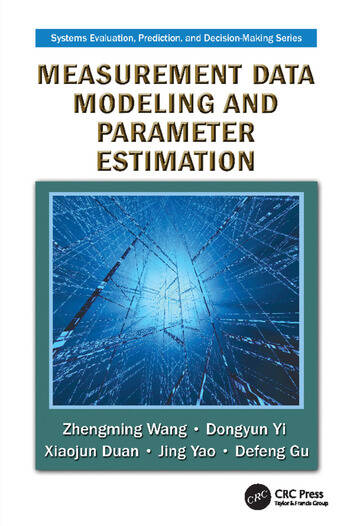Measurement Data Modeling and Parameter Estimation

1st Edition

Zhengming Wang, Dongyun Yi, Xiaojun Duan, Jing Yao, Defeng Gu

CRC Press
Published May 31, 2017
Reference - 553 Pages - 46 B/W Illustrations
ISBN 9781138114920 - CAT# K35393
Series: Systems Evaluation, Prediction, and Decision-Making

For Instructors Request Inspection Copy

was \$82.95

USD\$66.36

SAVE ~\$16.59

FREE Standard Shipping!

Preview

Summary

Measurement Data Modeling and Parameter Estimation integrates mathematical theory with engineering practice in the field of measurement data processing. Presenting the first-hand insights and experiences of the authors and their research group, it summarizes cutting-edge research to facilitate the application of mathematical theory in measurement and control engineering, particularly for those interested in aeronautics, astronautics, instrumentation, and economics.

Requiring a basic knowledge of linear algebra, computing, and probability and statistics, the book illustrates key lessons with tables, examples, and exercises. It emphasizes the mathematical processing methods of measurement data and avoids the derivation procedures of specific formulas to help readers grasp key points quickly and easily. Employing the theories and methods of parameter estimation as the fundamental analysis tool, this reference:

• Introduces the basic concepts of measurements and errors
• Applies ideas from mathematical branches, such as numerical analysis and statistics, to the modeling and processing of measurement data
• Examines methods of regression analysis that are closely related to the mathematical processing of dynamic measurement data
• Covers Kalman filtering with colored noises and its applications

Converting time series models into problems of parameter estimation, the authors discuss modeling methods for the true signals to be estimated as well as systematic errors. They provide comprehensive coverage that includes model establishment, parameter estimation, abnormal data detection, hypothesis tests, systematic errors, trajectory parameters, and modeling of radar measurement data. Although the book is based on the authors’ research and teaching experience in aeronautics and astronautics data processing, the theories and methods introduced are applicable to processing dynamic measurement data across a wide range of fields.

Instructors

We provide complimentary e-inspection copies of primary textbooks to instructors considering our books for course adoption.